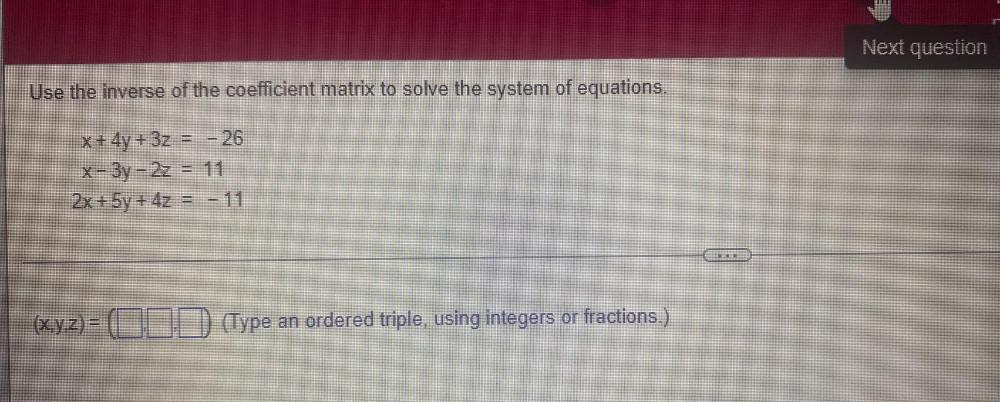Question:

# Use the inverse of the coefficient matrix to solve the system of equations. x + 4y + 3z = - 26 x - 3y - 2z = 11 2x + 5y + 4z = -11 (x,y,z)= (__,__,__)Use the inverse of the coefficient matrix to solve the system of equations. x + 4y + 3z = - 26 x - 3y - 2z = 11 2x + 5y + 4z = -11 (x,y,z)= (__,__,__)